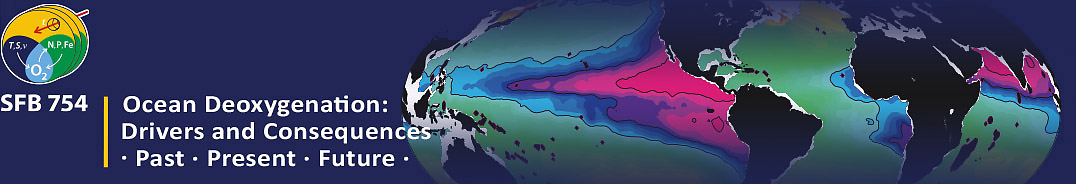#Ocean Deoxygenation Conference | Kiel 2018

3-7 September 2018
Audimax | Kiel University
Europe/Berlin timezone

## Hydroxylamine as a precursor for nitrous oxide in the equatorial Atlantic Ocean and eastern tropical South Pacific Ocean

Not scheduled
2h 30m
Audimax | Kiel University

#### Audimax | Kiel University

Christian-Albrechts-Platz 2 | 24118 Kiel | GERMANY
Poster 10 Biogeochemical Cycles: Feedbacks and Interactions

Frederike Korth

### Description

Hydroxylamine (NH$_{2}$OH), a short-term intermediate in the nitrogen cycle, is a potential precursor of nitrous oxide (N$_{2}$O) formation in the ocean. During nitrification, the oxidation of ammonium (NH$_{4}^{+}$) to nitrate (NO$_{3}^{-}$), N$_{2}$O is produced as a by-product via NH$_{2}$OH and nitrite (NO$_{2}^{-}$). However, measurements of NH$_{2}$OH in the ocean are sparse. Here, we present a dataset of depth profiles of NH$_{2}$OH from the equatorial Atlantic Ocean and the eastern tropical South Pacific (ETSP) and compare it to N$_{2}$O, NO$_{3}^{-}$ and NO$_{2}^{-}$ profiles under varying oxygen conditions. Overall, the NH$_{2}$OH concentrations were in the lower nanomolar range and lower than N$_{2}$O concentrations. In the equatorial Atlantic Ocean, where nitrification is the dominant N$_{2}$O pathway, stepwise multiple regressions demonstrated that N$_{2}$O, NH$_{2}$OH and NO$_{3}^{-}$ concentrations were highly correlated, indicating that NH$_{2}$OH is a precursor of N$_{2}$O and a tracer for nitrification. This relationship weakens in the ETSP and no correlations are found in the oxygen minimum zone (OMZ) off Peru where besides nitrification other N$_{2}$O pathways like denitrification occur.

Affiliation Geomar Postdoc Yes fkorth@geomar.de

### Primary authors

Frederike Korth Annette Kock Damian L. Arévalo-Martínez Hermann W. Bange

### Presentation Materials

There are no materials yet.